# 二叉树（Binary Tree）

/*   A simple binary tree
*        A ---------> A is root node
*       / \
*      /   \
*     B     C
*    /     / \
*   /     /   \
*   D     E    F ---> leaves: D, E, F
*
*       (1)      ---> Height: 3
*/


## 分类

/*  Complete Binary Tree (CBT) and Full Binary Tree (FBT)
*        A              A                A
*       / \            / \              / \
*      /   \          /   \            /   \
*     B     C        B     C          B     C
*    / \            / \   / \              / \
*   /   \          /   \ /   \            /   \
*   D    E        D    E F    G          D     E
*
*      (2)             (3)               (4)
*      CBT             FBT             not CBT
*/


(3)是一个满二叉树；

。所谓的“最后一层节点优先集中在左边”，用语言很难解释，但是结合上例的(2)和(4)可以很好理解。

# 二叉树的构建和遍历

## 节点定义

// A simple binary tree node define
typedef struct __TreeNode
{
int val;
struct __TreeNode *left, *right;
}TreeNode;


## 遍历

// root is in middle order travel, (1):D->B->A->E->C->F
void inorder(TreeNode *root)
{
if (root == NULL) return;
inorder(root->left);
printf("%d ",root->val); // visit
inorder(root->right);
}
// previous visit root order travel, (1):A->B->D->C->E->F
void preorder(TreeNode *root)
{
if (root == NULL) return;
printf("%d ",root->val); // visit
preorder(root->left);
preorder(root->right)
}
// post vist root order travel, (1):D->B->E->F->C->A
void postorder(TreeNode *root)
{
if (root == NULL) return;
postorder(root->left);
postorder(root->right);
printf("%d ",root->val); // visit
}


// level order travel, (1):A->B->C->D->E->F
void levelorder(TreeNode *root)
{
if(root==NULL) return;
queue<TreeNode*> q;
for(q.push(root); q.size(); q.pop()){
TreeNode *r = q.front();
printf("%d ",r->val);    // visit
if (r->left) q.push(r->left);
if (r->right) q.push(r->right);
}
}


# 二叉搜索树（Binary Search Tree）

## 概念

1. 其左子树（left subtree）下的每个后代节点（descendant node）的值都小于节点 n 的值；

2. 其右子树（right subtree）下的每个后代节点的值都大于节点 n 的值。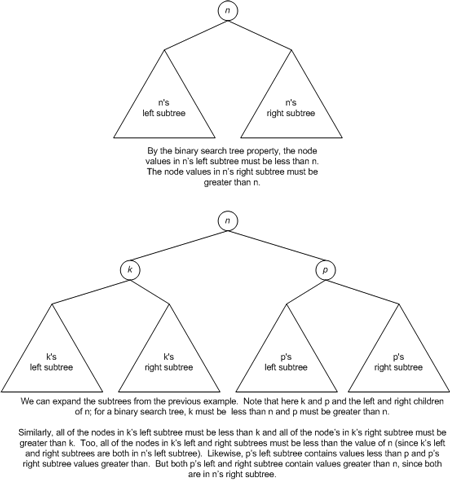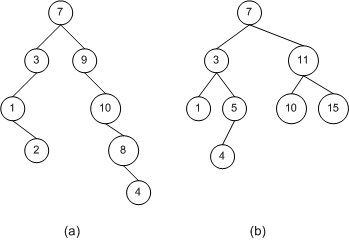## 查找算法

1. 如果 c 值为空，则 n 不在 BST 中；

2. 比较 c 和 n 的值；

3. 如果值相同，则找到了指定节点 n；

4. 如果 n 的值小于 c，那么如果 n 存在，必然在 c 的左子树中。回到第 1 步，将 c 的左孩子作为 c；

5. 如果 n 的值大于 c，那么如果 n 存在，必然在 c 的右子树中。回到第 1 步，将 c 的右孩子作为 c；

## 复杂度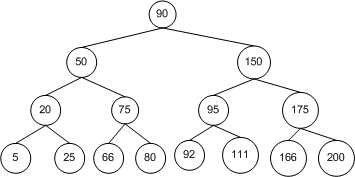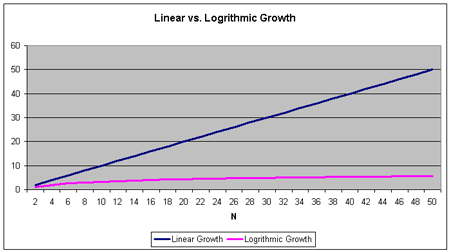### 最差情况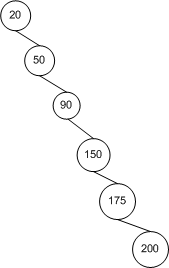## 插入节点

1. 如果节点 c 为空，则节点 c 的父节点将作为节点 n 的父节点。如果节点 n 的值小于该父节点的值，则节点 n 将作为该父节点的左孩子；否则节点 n 将作为该父节点的右孩子。

2. 比较节点 c 与节点 n 的值。

3. 如果节点 c 的值与节点 n 的值相等，则说明用户在试图插入一个重复的节点。解决办法可以是直接丢弃节点 n，或者可以抛出异常。

4. 如果节点 n 的值小于节点 c 的值，则说明节点 n 一定是在节点 c 的左子树中。则将父节点设置为节点 c，并将节点 c 设置为节点 c 的左孩子，然后返回至第 1 步。

5. 如果节点 n 的值大于节点 c 的值，则说明节点 n 一定是在节点 c 的右子树中。则将父节点设置为节点 c，并将节点 c 设置为节点 c 的右孩子，然后返回至第 1 步。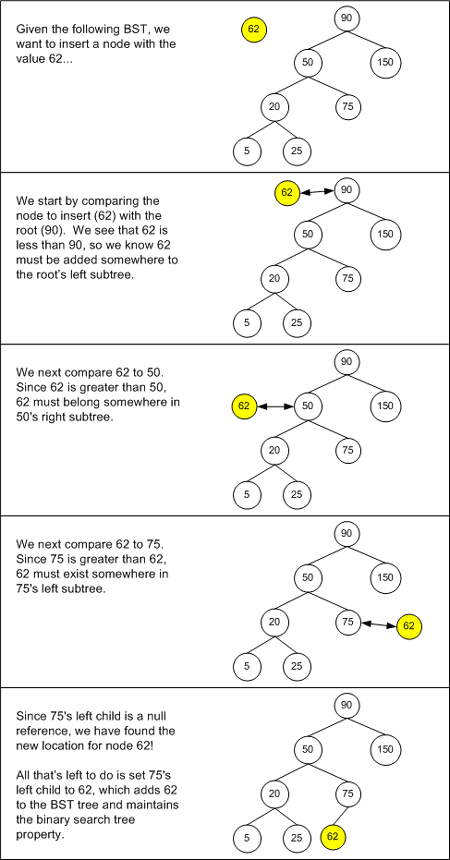BST 的插入算法的复杂度与查找算法的复杂度是一样的：最佳情况是 O(log­2n)，而最坏情况是 O(n)。因为它们对节点的查找定位策略是相同的。

## 删除节点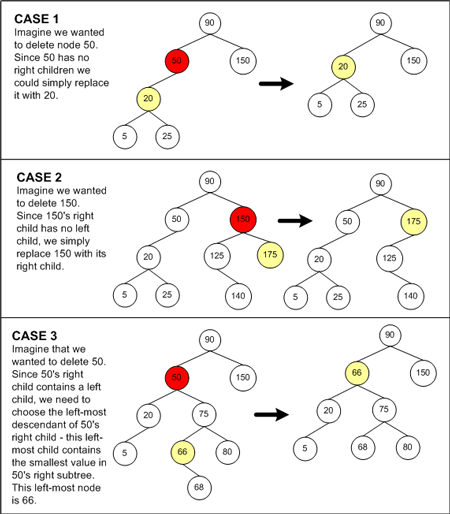## 遍历节点

• 前序遍历（Perorder traversal）

• 中序遍历（Inorder traversal）

• 后序遍历（Postorder traversal）### 前序遍历（Perorder traversal）

• 访问节点 c；

• 对节点 c 的左孩子重复第 1 步；

• 对节点 c 的右孩子重复第 1 步；

### 中序遍历（Inorder traversal）

• 访问节点 c 的左孩子；

• 对节点 c 重复第 1 步；

• 对节点 c 的右孩子重复第 1 步。

### 后序遍历（Postorder traversal）

• 访问节点 c 的左孩子；

• 对节点 c 的右孩子重复第1 步；

• 对节点 c 重复第 1 步；

## 其他

// Asuming each node value is not equal
/*  A simple binary search tree
*           6                  6
*          / \                / \
*         /   \              /   \
*        3     8            3     8
*       /     / \          /     / \
*      /     /   \        /     /   \
*     2     7     9      2     4*    9
*
*       (A) BST             (B) Not BST
*/


// Initialize a bst
TreeNode *bst_init(int arr[], int n)
{
if (n<1) return NULL;
TreeNode *r = (TreeNode*)malloc(sizeof(TreeNode));
r->val = arr;     // ensure bst_append will not update root address
r->left = r->right = NULL;
for (; --n; bst_append(r,arr[n]));
return r;
}


// Append a node to bst, return add count
int bst_append(TreeNode *r, int val)
{
// find insertion position
for (; r && r->val!=val;){
if (r->val < val && r->right) r=r->right;
else if (r->val > val && r->left) r=r->left;
else break;
}
if (r==NULL || r->val==val) return 0;
TreeNode *tn = (TreeNode*)malloc(sizeof(TreeNode));
tn->left = tn->right = NULLL;
tn->val = val;
if (r->val < val) r->right = tn;
else r->left = tn;
return 1;
}


// Find value in bst, return node address
TreeNode *bst_find(TreeNode *r, int val)
{
for (; r && r->val!=val;){
if (r->val < val) r=r->right;
else if (r->val > val) r=r->left;
}
return r;
}


/* Erase node from a bst - sketch, i' is special for erase 6 (i)
*       6            d=6,(3)       f=6           6           d=6,(5)
*      / \            / \          / \          / \           /  \
*     /   \          /   \        /   \        /   \         /    \
*    3    8        p=3    8     d=3    8      3   f=8      f=3     8
*   /    / \        /    / \     /    / \     /    / \      / \   / \
*  /    /   \      /    /   \   /    /   \   /    /   \    /   \ /   \
*  2    7    9    2    7    9   2    7    9  2   d=7  9   2  p=5 7   9
*                                                             /
*     BST             (i)           (ii)        (iii)        /  (i')
*                   erase 6      erase 3      erase 7       4
*/


(i) 待删除的节点有两个子节点：以删除6为例，为了便于说明，这里将待删除节点称为d=6，其前驱节点为p=3。按照(i)图示方法，可以将其前驱节点p的值替换待删除节点d，并删除前驱节点。注意，如果前驱节点p仍有子节点（子树），则其必然是左节点（左子树），为什么？请自行思考。这里将前驱节点p的父节点称为f，此时的f正好是d，但不是所有情况都是。对于(i’)图示，前驱节点p=5的父节点为f=3，当删除d=6时，可以将f的右子节点指向p的左子节点；对于(i)，由于f与d相同，所以可以直接将d的左子节点指向p的左子节点。

(ii)待删除的节点有一个子节点：以删除3为例，由于只有一个子节点，所以可将d节点的子节点继承d，此时需要将d的父节点f=6的子节点指向继承节点。并且需要区分当前删除节点d是父节点f的左子节点还是右子节点，以及d节点的子节点是左子还是右子。图示d为f的左子节点，d有左子节点，所以将f的左子节点指向d的左子节点。

(iii)待删除的节点无子节点：以删除7为例，很简单，将其直接删除，并且将其父节点f的子节点指向空。同样需要判断d是f的左子还是右子。

int bst_erase(TreeNode *r, int val)
{
TreeNode *f, *p, *d;
// f is father node
// p is precursor node
// d is to be deleted node
for (f=NULL,d=r; d && d->val!=val;){
f = d;
if (d->val < val) d=d->right;
else d=d->left;
}
if (d==NULL) return 0;          // cannot find erase node

if (d->left && d->right){     // deletion has two children
// find deletion node d's precursor
for (f=d,p=d->left; p->right; f=p, p=p->right);
d->val = p->val;          // replace deletion val by precursor
if (f==d) d->left = p->left;// case (i)
else f->right = p->left;  // case (i')
}
else if (d->left==NULL && d->right==NULL){
if (d==r) return -1;        // deletion is single root, this will
// deal this at calling procedure.
// deletion is leaf
if (f->left == d) f->left=NULL;
else if (f->right == d) f->right=NULL;
free(d);
}
else {  // deletion has single child node or branch
p = (d->left ? d->left : d->right);
d->val = p->val;
d->left = p->left;
d->right = p->right;
free(p);
}
return 1;   // return erase node count
}


# 二叉树问题

## 1. build123（）

    2
/ \
1   3


## 4. minValue（）

maxValue（）函数在结构上与此函数非常相似。

## 5. printTree（）

    4
/ \
2 5
/ \
1 3


## 6. printPostorder（）

    4
/ \
2 5
/ \
1 3


## 7. hasPathSum（）

      5
/ \
4   8
/   / \
11  13  4
/  \      \
7    2      1


Root-to-leaf paths:

path 1: 5 4 11 7
path 2: 5 4 11 2
path 3: 5 8 13
path 4: 5 8 4 1


## 9. mirror() 镜像

    4
/ \
2 5
/ \
1 3


  4
/ \
5 2
/ \
3 1


## 10. doubleTree（）

  2
/ \
1   3


       2
/ \
2   3
/   /
1   3
/
1


## 二进制搜索树检查（针对问题13和14）

a.  5   -> TRUE
/ \
2   7

b.  5   -> FALSE, because the 6 is not ok to the left of the 5
/ \
6   7

c.   5  -> TRUE
/ \
2   7
/
1

d.   5  -> FALSE, the 6 is ok with the 2, but the 6 is not ok with the 5
/ \
2   7
/ \
1   6


## 14. isBST（）-版本2

min和max的初始值应为INT_MIN和INT_MAX-从那里开始变窄。

/*
Returns true if the given tree is a binary search tree
(efficient version).
*/
int isBST2(struct node* node) {
return(isBSTRecur(node, INT_MIN, INT_MAX));
}

/*
Returns true if the given tree is a BST and its
values are >= min and <= max.
*/
int isBSTRecur(struct node* node, int min, int max) {


## 15. Tree-List 树状列表

Tree-List 问题是有史以来最大的递归指针问题之一，它也恰好使用二叉树。

CLibarary＃109 http://cslibrary.stanford.edu/109/详细研究了Tree-List问题，并包括C和Java解决方案代码。

# Java二叉树和解决方案

Node类是私有的-仅用于BinaryTree内部的内部存储，并且不公开给客户端。

# 二叉树的序列化（serialize）和反序列化（deserialize）

## 概念

/*  A simple binary tree four typical traversals
*           A
*          / \        in order   : D->B->A->E->C->F
*         /   \       pre order  : A->B->D->C->E->F
*        B     C      post order : D->B->E->F->C->A
*       /     / \     level order: A->B->C->D->E->F
*      /     /   \
*     D     E     F
*
*          (1)
*/


// Using In order and Pre order to deserialize
/*
*        A*               A              A             A
*       / \    ====>     / \            / \           / \
*      /   \            /   \          /   \         /   \
*    D-B  E-C-F        B*  E-C-F      B   E-C-F     B    C*
*                     / \            /             /    / \
*                    /   \          /             /    /   \
*                   D    NULL      D*             D   E     F
*         root         root       root             root
*          |             |          |               |
*  IN: D-B-A-E-C-F     D-B          D             E-C-F
*  PRE:A-B-D-C-E-F     B-D-C-E-F    D-C-E-F       C-E-F
*      |               |            |             |
*     root           root          root          root
*/


// Using In order and Pre order to deserialize
TreeNode *deserialize(int pre[], int in[], int n, int begin, int end)
{
static int id = 0;              // current position in PRE order
if (begin==0 && end==n) id=0;   // reset id
TreeNode *r = (TreeNode*)malloc(sizeof(TreeNode));
int pos;                        // current root position in IN order
for (pos=begin; pos<end && in[pos]!=pre[id]; ++pos);
if (in[pos]!=pre[id]) exit(-1); // preorder or inorder is error
r->val = pre[id++];
r->left = deserialize(pre,in,n,begin,pos);
r->right= deserialize(pre,in,n,pos+1,end);
return r;
}


n 为节点总数，也即为数组的长度；start和end表示寻找中序遍历的区间范围 [start,end)。

## 核心问题

1、 如何判断每个节点应该在第几行输出，或者说输出的时候如何判断该换行？

2、 如何安排节点的位置，使左右孩子在其父节点的两边，以便直观地看出各个节点之间的关系？

3、 因为没有使用图形库，不能在任意位置输出，所以输出必须是一次性的，也就是在输出前就得确定图中所有字符应在的位置。

4、 通用性：

## 解决思路

### 第几行

324

71， 776

43， 159， 425， 817

389


@Override
public void print() {
TreeNode<V> node = root;
int[] levelArray = new int;
levelArray = 1;
// 临时存放元素的列表
List<V> tempList = new ArrayList<>();
int level = 0;
while (!queue.isEmpty()) {
node = queue.poll();
if (node.getLeft() != null) {
levelArray[level+1]++;
}
if (node.getRight() != null) {
levelArray[level+1]++;
}
// 判断是否为当前这行最后一个元素
if(tempList.size() == levelArray[level]) {
// 输出
System.out.println(tempList);
tempList.clear();
level++;
}
}
}


@Test
public void printTest() {
BinarySearchTree<Integer> tree = new BinarySearchTree<>();

tree.print();
}



[2, 7]
[1, 3, 6, 9]


## 如何确定每一个元素的位置？

BinarySearchTree<Integer> tree = new BinarySearchTree<>();

System.out.println(tree.inOrder());
System.out.println(tree.levelOrder());


[1, 2, 3, 5, 6, 7, 9]
[5, 2, 7, 1, 3, 6, 9]


package com.github.houbb.data.struct.core.util.tree.component;

/**
* @author binbin.hou
* @since 1.0.0
*/
public class PrintTreeNode<V extends Comparable<? super V>> {

private V data;

/**
* 左节点
*/
private boolean isLeft;

/**
* 右节点
*/
private boolean isRight;

/**
* 是否为最后一个元素
*/
private boolean isEndLine;

/**
* 当前层级 y 轴偏移量
*/
private int level;

/**
* x 轴的偏移量
*/
private int offset;

//fluent setter & getter

}


/**
* 打印思路
*/
@Override
public void print() {
List<PrintTreeNode<V>> printList = new ArrayList<>();
int level = 0;
TreeNode<V> node = root;
//root
PrintTreeNode<V> printTreeNode = buildPrintNode(node, level, false, false, true);
// 入队的时候构建元素
int[] levelArray = new int;
levelArray[level] = 1;
// 临时存放元素的列表
List<V> tempList = new ArrayList<>();
while (!queue.isEmpty()) {
node = queue.poll();
if (node.getLeft() != null) {
levelArray[level+1]++;
PrintTreeNode<V> leftNode = buildPrintNode(node.getLeft(), level+1,
true, false, false);
}
if (node.getRight() != null) {
levelArray[level+1]++;
PrintTreeNode<V> rightNode = buildPrintNode(node.getRight(), level+1,
false, true, false);
}
// 判断是否为当前这行最后一个元素
if(tempList.size() == levelArray[level]) {
printList.get(printList.size()-1).endLine(true);
tempList.clear();
level++;
}
}
// 中序遍历，确定 x 坐标
List<V> inOrders = inOrder();
for(PrintTreeNode<V> node1 : printList) {
V value = node1.data();
int index = inOrders.indexOf(value);
node1.offset(index);
}
// 输出
int offset = 0;
for(PrintTreeNode<V> node1 : printList) {
int xOffset = node1.offset();
String text = leftPad(xOffset, offset, node1.data());
offset += text.length();
System.out.print(text);
if(node1.endLine()) {
System.out.println();
offset = 0;
}
}
}

private String leftPad(int xoffset, int offset, V value) {
int left = xoffset - offset;
if(left <= 0) {
return value.toString();
}
// 直接填充
return CharUtil.repeat(' ', left)+value.toString();
}


BinarySearchTree<Integer> tree = new BinarySearchTree<>();

tree.print();


   5
2   7
1 3 6 9


（1）没有比较直观的 /\ 符号链接，需要自行脑补。

（2）如果元素重复，可能导致位置错乱。这个是可以改进的。

# 参考资料

http://cslibrary.stanford.edu/110/BinaryTrees.html

https://www.javatpoint.com/binary-tree

https://www.javatpoint.com/tree

[数据结构]——二叉树（Binary Tree）、二叉搜索树（Binary Search Tree）及其衍生算法

【Java】 二叉树的遍历（递归与循环+层序遍历）